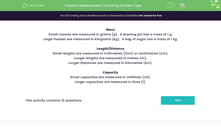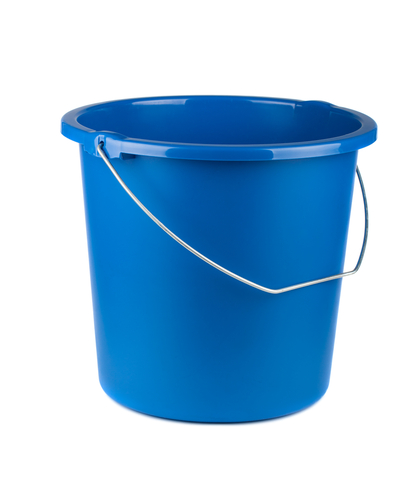# Select the Most Appropriate Unit of Measure

In this worksheet, students will select the most sensible measurement or unit of measurement for the given object.Key stage:  KS 2

Curriculum topic:   Measurement

Curriculum subtopic:   Use Different Measures

Difficulty level:#### Worksheet Overview

Mass

Small masses are measured in grams (g)

A drawing pin has a mass of 1 g.

Large masses are measured in kilograms (kg)

A bag of sugar has a mass of 1 kg.

Length/Distance

Small lengths are measured in millimetres (mm) or centimetres (cm)

We would measure the length of an ant in mmWe would measure the length of a book in cmLonger lengths are measured in metres (m)

We would measure the length of a classroom in mLonger distances are measured in kilometres (km)

We would measure the distance between towns in km

Capacity

Small capacities are measured in millilitres (ml)

We would measure a glass of milk in mlLarger capacities are measured in litres (l)

We would measure a bucket of water in lIn this activity, we will use the information on this page to answer a variety of questions.

We can look at this page again at any point by clicking on the red help button that will appear on the screen.

Good luck!### What is EdPlace?

We're your National Curriculum aligned online education content provider helping each child succeed in English, maths and science from year 1 to GCSE. With an EdPlace account you’ll be able to track and measure progress, helping each child achieve their best. We build confidence and attainment by personalising each child’s learning at a level that suits them.

Get started